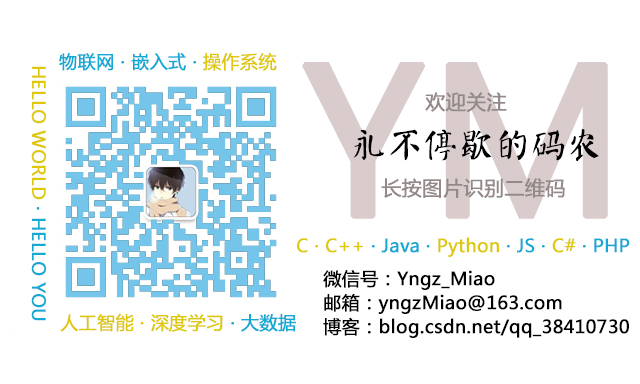• 如果你使用过python，你会发现字符串和int/float/double便捷的拼接方式；...通过std::to_string()将数字类型转换成std::string类型，从而可以直接使用+完成字符串拼接。 # include <iostream> i...
如果你使用过python，你会发现字符串和int/float/double便捷的拼接方式；但如果你使用C++，可能你每次需要的时候搜索一下才能知道。本文提供两种简单的方式来完成这个功能。
本文实例源码github地址：https://github.com/yngzMiao/yngzmiao-blogs/tree/master/2019Q4/20191127。

std::to_string()
通过std::to_string()将数字类型转换成std::string类型，从而可以直接使用+完成字符串的拼接。
# include <iostream>

int main(int argc, char const *argv[])
{
std::string str = "hello " + std::to_string(1);
std::cout << str << std::endl;

return 0;
}

需要注意的是，std::string是C++11才有的方法，在g++编译的时候需要指定-std=c++11。
编译并运行这段程序：
yngzmiao@yngzmiao-virtual-machine:~/test$g++ test.cpp -o test -std=c++11 yngzmiao@yngzmiao-virtual-machine:~/test$ ./test
hello 1

同时，如果想要转化为const char*的类型，可以使用c_str()方法：
std::string str = "hello 1";
str.c_str();

如果想要去除const属性，需要使用到const_cast：
const char* const_char_str = str.c_str();
std::cout << const_char_str << std::endl;

char* char_str = const_cast<char*>(const_char_str);
std::cout << char_str << std::endl;


ostringstream
通过字符串流来完成字符串和数字的拼接，再将字符串流的内容转化为std::string的类型。
使用ostringstream之前，需要指定头文件：
# include <iostream>
# include <sstream>

int main(int argc, char const *argv[])
{
std::ostringstream oss;
oss << "hello " << 2;
std::string str = oss.str();
std::cout << str << std::endl;

return 0;
}

编译并运行这段程序：
yngzmiao@yngzmiao-virtual-machine:~/test$g++ test.cpp -o test -std=c++11 yngzmiao@yngzmiao-virtual-machine:~/test$ ./test
hello 2

使用ostringstream，如何来清空内容呢？
oss.str("");

对float小数点也可以进行一些格式化：
oss.setf(std::ios::fixed);				//小数定点化
oss.precision(2);						//小数点后2位

需要注意的是，使用小数点的格式化需要在传入小数之前来完成，否则不会生效。例如：
oss << "hello " << 3.14159265357;
oss.setf(std::ios::fixed);
oss.precision(2);

oss.setf(std::ios::fixed);
oss.precision(2);
oss << "hello " << 3.14159265357;

相比较来说，后者会保留小数点后两位，而前者不会。

注意点
这两种方式在linux g++11版本上编译一般都不会出现问题，但是在Android NDK上编译，第一种方式可能会出现问题，因此此时建议使用第二种方式。展开全文• 使用这种写法，Python 会自动将两个字符串拼接在一起。 【示例】以连续书写的形式拼接字符串： str1 = "Python教程" "http://c.biancheng.net/python/" print(str1) str2 = "Java" "Python" "C++
在 Python 中拼接（连接）字符串很简单，可以直接将两个字符串紧挨着写在一起，具体格式为：
strname = “str1” “str2”
strname 表示拼接以后的字符串变量名，str1 和 str2 是要拼接的字符串内容。使用这种写法，Python 会自动将两个字符串拼接在一起。
【示例】以连续书写的形式拼接字符串：
str1 = "Python教程" "http://www.baidu.com/python/"
print(str1)
str2 = "Java" "Python" "C++" "PHP"
print(str2)

运行结果：
Python教程http://www.baidu.com/python/
JavaPythonC++PHP

需要注意的是，这种写法只能拼接字符串常量。
如果需要使用变量，就得借助+运算符来拼接，具体格式为：
strname = str1 + str2
当然，+运算符也能拼接字符串常量。
【示例】使用+运算符拼接字符串：
name = "C++教程"
url = "http://www.baidu.com/cplus/"
info = name + "的网址是：" + url
print(info)

运行结果：
C++教程的网址是：http://www.baidu.com/cplus/

Python字符串和数字的拼接
在很多应用场景中，我们需要将字符串和数字拼接在一起，而 Python 不允许直接拼接数字和字符串，所以我们必须先将数字转换成字符串。可以借助 str() 和 repr() 函数将数字转换为字符串，它们的使用格式为：
str(obj)
repr(obj)
obj 表示要转换的对象，它可以是数字、列表、元组、字典等多种类型的数据。
请看下面的代码：
name = "C语言中文网"
age = 8
course = 30
info = name + "已经" + str(age) + "岁了，共发布了" + repr(course) + "套教程。"
print(info)

运行结果：
C语言中文网已经8岁了，共发布了30套教程。
str() 和 repr() 的区别
str() 和 repr() 函数虽然都可以将数字转换成字符串，但它们之间是有区别的：

str() 用于将数据转换成适合人类阅读的字符串形式。
repr() 用于将数据转换成适合解释器阅读的字符串形式（Python 表达式的形式），适合在开发和调试阶段使用；如果没有等价的语法，则会发生 SyntaxError 异常。

请看下面的例子：
s = "http://www.baidu.com/shell/"
s_str = str(s)
s_repr = repr(s)
print( type(s_str) )
print (s_str)
print( type(s_repr) )
print (s_repr)

运行结果：
<class ‘str’>
http://www.baidu.com/shell/
<class ‘str’>
‘http://www.baidu.com/shell/’
本例中，s 本身就是一个字符串，但是我们依然使用 str() 和 repr() 对它进行了转换。从运行结果可以看出，str() 保留了字符串最原始的样子，而 repr() 使用引号将字符串包围起来，这就是 Python 字符串的表达式形式。
另外，在 Python 交互式编程环境中输入一个表达式（变量、加减乘除、逻辑运算等）时，Python 会自动使用 repr() 函数处理该表达式。


展开全文• 在 Python 中拼接（连接）字符串很简单，可以直接将两个字符串紧挨着写在一起，具体格式为：...使用这种写法，Python 会自动将两个字符串拼接在一起。【示例】以连续书写的形式拼接字符串：str1 = "Pytho...
在 Python 中拼接（连接）字符串很简单，可以直接将两个字符串紧挨着写在一起，具体格式为：strname = "str1" "str2"strname 表示拼接以后的字符串变量名，str1 和 str2 是要拼接的字符串内容。使用这种写法，Python 会自动将两个字符串拼接在一起。【示例】以连续书写的形式拼接字符串：str1 = "Python教程" "http://c.biancheng.net/python/"print(str1)str2 = "Java" "Python" "C++" "PHP"print(str2)运行结果：Python教程http://c.biancheng.net/python/JavaPythonC++PHP需要注意的是，这种写法只能拼接字符串常量。如果需要使用变量，就得借助+运算符来拼接，具体格式为：strname = str1 + str2当然，+运算符也能拼接字符串常量。【示例】使用+运算符拼接字符串：name = "C++教程"url = "http://c.biancheng.net/cplus/"info = name + "的网址是：" + urlprint(info)运行结果：C++教程的网址是：http://c.biancheng.net/cplus/Python字符串和数字的拼接在很多应用场景中，我们需要将字符串和数字拼接在一起，而 Python 不允许直接拼接数字和字符串，所以我们必须先将数字转换成字符串。可以借助 str() 和 repr() 函数将数字转换为字符串，它们的使用格式为：str(obj)repr(obj)obj 表示要转换的对象，它可以是数字、列表、元组、字典等多种类型的数据。请看下面的代码：name = "C语言中文网"age = 8course = 30info = name + "已经" + str(age) + "岁了，共发布了" + repr(course) + "套教程。"print(info)运行结果：C语言中文网已经8岁了，共发布了30套教程。str() 和 repr() 的区别str() 和 repr() 函数虽然都可以将数字转换成字符串，但它们之间是有区别的：str() 用于将数据转换成适合人类阅读的字符串形式。repr() 用于将数据转换成适合解释器阅读的字符串形式（Python 表达式的形式），适合在开发和调试阶段使用；如果没有等价的语法，则会发生 SyntaxError 异常。请看下面的例子：s = "http://c.biancheng.net/shell/"s_str = str(s)s_repr = repr(s)print( type(s_str) )print (s_str)print( type(s_repr) )print (s_repr)运行结果：http://c.biancheng.net/shell/"http://c.biancheng.net/shell/"本例中，s 本身就是一个字符串，但是我们依然使用 str() 和 repr() 对它进行了转换。从运行结果可以看出，str() 保留了字符串最原始的样子，而 repr() 使用引号将字符串包围起来，这就是 Python 字符串的表达式形式。另外，在 Python 交互式编程环境中输入一个表达式（变量、加减乘除、逻辑运算等）时，Python 会自动使用 repr() 函数处理该表达式。
展开全文• 一、数字字符串 头文件 #include<string> #include<typeinfo> 1.1 int型数字字符串 int num = 123; string num2str = to_string(num); cout << typeid(to_string(num) == typeid(string) <...
文章目录一、num转string1.1 int型数字转字符串1.2 float/double型数字转字符串（不补0）二、string转num2.1 使用stringstream类处理2.2 使用\处理三、char[]转num四、char[]与string的相互转换五、字符串拼接5.1 string + string5.1 string + char*参考文献
一、num转string
头文件
#include<string>
#include<typeinfo>

1.1 int型数字转字符串
int num = 123;
string num2str = to_string(num);
cout << typeid(to_string(num) == typeid(string) << endl;  // true

1.2 float/double型数字转字符串（不补0）
头文件
#include<sstream>

double num = 123.56;  // float同理
stringstream sstream;
sstream << num;
string num2str = sstream.str();  // num2str = "123.56"
cout << typeid(sstream.str() == typeid(string) << endl;  // true
sstream.clear();  // 若在用一个流中处理大量数据，则需手动清除缓存，小数据或不同流可忽略

缺点：处理大量数据转换速度较慢。stringstream不会主动释放内存，如果要在程序中用同一个流，需要适时地清除一下缓存，用stream.clear()
二、string转num
2.1 使用stringstream类处理

字符串转int/float/double型数字（不补0）

string str = "456.78";
double num;        // float同理,int需要str为整数,否则报错
stringstream sstream(str);
sstream >> num;    // num = 456.78
cout << typeid(num == typeid(double) << endl;  // true

2.2 使用<string>处理
头文件
#include<string>

string str = "456.78";
double num = stod(str);   // num = 456.78
cout << typeid(num == typeid(double) << endl;  // true

下面给出常用的转换方法，完整转换方法请见《C++中的字符串(String)和数值转换》

转换数字的类型
默认
完整参数
功能
全参例子

int
stoi(s)
stoi(s,p,b)
把字符串s从p开始转换成b进制的int
stoi(s, 0, 10)

float
stof(s)
stof(s,p)
把字符串s从p开始转换成float

double
stod(s)
stod(s,p)
把字符串s从p开始转换成double

long
stol(s)
stol(s,p,b)
把字符串s从p开始转换成b进制的long
stol(s, 0, 10)

三、char[]转num
头文件
#include<cstdio>

char ch = "-456.78";
// 注：atof(ch)只返回double数字，因此需要float可以自行转换成float
double num = atof(ch);   // num = -456.78
cout << typeid(num == typeid(double) << endl;  // true

下面给出常用的转换方法，完整转换方法请见《C++中的字符串(String)和数值转换》

转换数字的类型
默认
功能

int
atoi(s)
将字符串s[n]转换为整型值

double
atof(s)
将字符串s[n]转换为double

long
atol(s)
将字符串s[n]转换为long

四、char[]与string的相互转换

4.1 字符数组char[]转换string（直接赋值即可）

char ch = "Hellow World";
string str = ch;  // str = "Hellow World"
cout << typeid(str == typeid(string) << endl;  // true


4.2 字符数组string转换char[]

string str = "Hellow World";
char ch = {0};
for (int i=0;i < str.length();i++)
ch[i] = str[i];
cout << ch << endl;  // ch = "Hellow World"

五、字符串拼接
5.1 string + string
string str1 = "aaa";
strint str2 = "bbb";
cout << str1 + str2 << endl; // "aaabbb"
cout << str1 + "bbb" << endl; // "aaabbb"

5.1 string + char*
string str1 = "aaa";
char* str2 = "bbb";
cout << str1 + str2 << endl; // "aaabbb"

持续积累中~
参考文献
 C++ 字符串与字符数组详解
 C++中的字符串(String)和数值转换


展开全文• 方法一： #include &lt;iostream&gt; #include &lt;string&gt; #include &lt;sstream&gt; using namespace std; int main() {  int a = 2; ... a ...
• int a = 2; string b = "xyz"; char buf ; sprintf( buf, %d, i ); string c = buf+b;...

# c++字符串拼接数字c++ 订阅